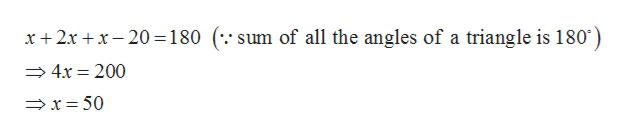# One angle of a triangle is two times as large as another. The measure of the third angle is 20 degrees less than that of the smallest angle. Find the measure of each angle.

Question
9 views

One angle of a triangle is two times as large as another. The measure of the third angle is 20 degrees less than that of the smallest angle. Find the measure of each angle.

check_circle

star
star
star
star
star
1 Rating
Step 1

Let x be the smallest angle of the triangle.

Step 2

Then according to given conditions, the other two angles are

Step 3

Now by using angle sum propert...help_outlineImage Transcriptionclose20180 (.' sum of all the angles of a triangle is 180 x2x 4x 200 x50 fullscreen

### Want to see the full answer?

See Solution

#### Want to see this answer and more?

Solutions are written by subject experts who are available 24/7. Questions are typically answered within 1 hour.*

See Solution
*Response times may vary by subject and question.
Tagged in

### Other